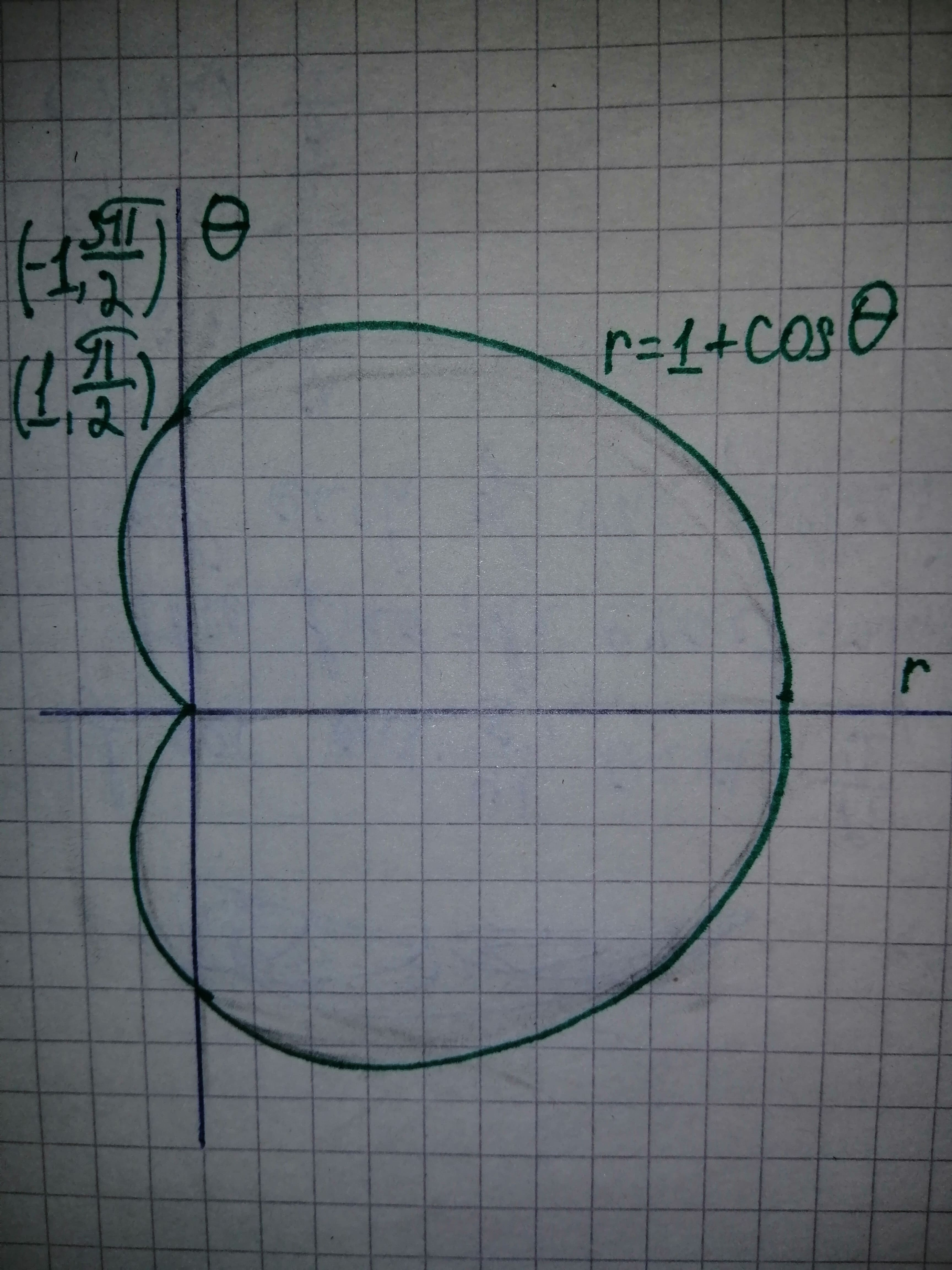# \displaystyle{\left(-{1},\frac{{{3}\pi}}{{2}}\right)} lies on the polar graphCheyanne Leigh 2021-02-26 Answered

The reason ehy the point $\left(-1,\frac{3\pi }{2}\right)$ lies on the polar graph $r=1+\mathrm{cos}\theta$ even though it does not satisfy the equation.

You can still ask an expert for help

• Questions are typically answered in as fast as 30 minutes

Solve your problem for the price of one coffee

• Math expert for every subject
• Pay only if we can solve itcrocolylec

The given equation of the polar curve is $r=1+\mathrm{cos}\theta .$
By substituing the point $\left(-1,\frac{3\pi }{2}\right)\in r=1+\mathrm{cos}\theta$
$r=1+\mathrm{cos}\theta$
$-1=1+\mathrm{cos}\left(\frac{3\pi }{2}\right)$
$-1=1+0$
$-1\ne 1$
Therefore, the point $\left(-1,\frac{3\pi }{2}\right)$ does not satisfy the equation of the polar curve $r=1+\mathrm{cos}\theta .$
Use online graphing calculator and draw the graph of $r=1+\mathrm{cos}\theta$ as shown below in Figure 1 and identify the point $\left(-1,\frac{3\pi }{2}\right).$From Figure 1 it can be noted that the point $\left(-1,\frac{3\pi }{2}\right)$ lies on the graph.
Consider the point $\left(-1,\frac{3\pi }{2}\right)$ and change its radial coordinate to 1 and subtract $\pi$ from the directional coordinate to obtain the alternate representation of the point $\left(-1,\frac{3\pi }{2}\right).$
Therefore, the point $\left(-1,\frac{3\pi }{2}\right)$ can also be represented by $\left(1,\frac{3\pi }{2}-\pi \right)=\left(1,\frac{\pi }{2}\right).$
By substituing the point $\left(1,\frac{\pi }{2}\right)\in r=1+\mathrm{cos}\theta ,$
$r=1+\mathrm{cos}\theta$
$1=1+\mathrm{cos}\left(\frac{\pi }{2}\right)$
$1=1+0$
$1=1$
Thus, the point $\left(1,\frac{\pi }{2}\right)$ satisfies the polar equation $r=1+\mathrm{cos}\theta .$
And from Figure 1 it can be seen that the points $\left(1,\frac{\pi }{2}\right)\phantom{\rule{1em}{0ex}}\text{and}\phantom{\rule{1em}{0ex}}\left(-1,\frac{3\pi }{2}\right)$ are the same points.
Therefore, due to this multiple identity, the point $\left(-1,\frac{3\pi }{2}\right)$ lies on the graph even if does not satisfy the polar equation $r=1+\mathrm{cos}\theta$